## Extract all words but first or last from a cell in Excel

In this tutorial, we will learn how to use formulas to extract all words from a cell except the first or last one in Excel.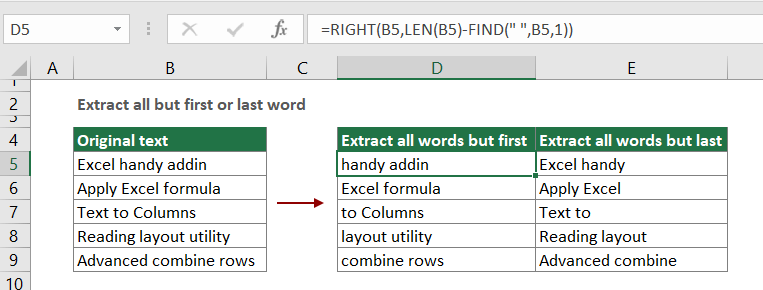Extract all words but first from a cell in Excel
Extract all words but last from a cell in Excel

#### Extract all words but first from a cell in Excel

As the below screenshot shown, you want to extract all words from each cell in column B except the first one, you can use a combination of the RIGHT, LEN and FIND functions to achieve it. Please do as follows.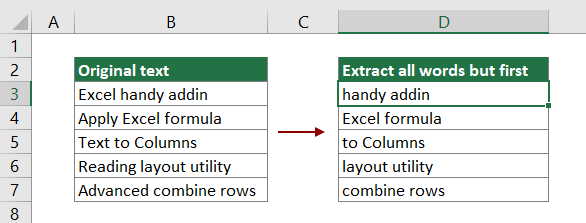Generic formula

=RIGHT(text_string,LEN(text_string)-FIND(" ",text_string,1))

Arguments

Text_string: The text string you will extract all words from it except the first one. It can be:

• The text string enclosed in quotation marks;
• Or a reference to a cell containing the text string.

How to use this formula?

1. Select a blank cell to output the extracted words. In this case, I select cell D3.

2. Enter the below formula into it and press the Enter key. And then select and drag the formula cell all the way down to apply it to other cells.

=RIGHT(B3,LEN(B3)-FIND(" ",B3,1))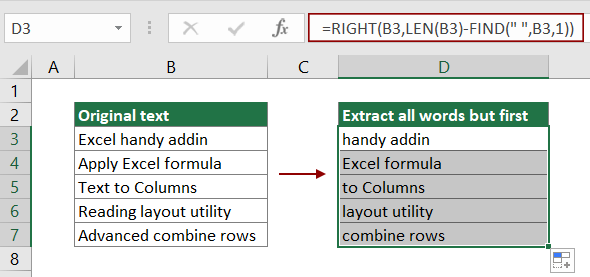Now all words in specific cells are extracted except the first words.

Note: In this above formula, B3 is the cell contains the words you will extract except the first one. Please change it based on your need.

How this formula works?

=RIGHT(B3,LEN(B3)-FIND(" ",B3,1))

1. LEN(B3): The LEN function calculates the total length of Excel handy addin and returns the result as 17;

2. FIND(" ",B3,1): As the words are separated by space, here the FIND function locates the position of the first space in Excel handy addin and returns the result as 6;

3. RIGHT(B3,17-6): The RIGHT function extracts 11 characters (17-6=11) from the right side of the text string "Excel handy addin". The result is "handy addin".

#### Extract all words but last from a cell in Excel

In this section, we are going to use a combination of the LEFT, FIND, SUBSTITUTE and the LEN functions to extract all words except the last one from a specific cell in Excel.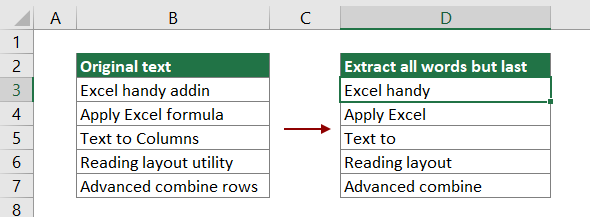Generic formula

=LEFT(text_string,FIND("[",SUBSTITUTE(text_string," ","[",LEN(text_string)-LEN(SUBSTITUTE(text_string," ",""))))-1)

Arguments

Text_string: The text string you will extract all words from it except the last one. It can be:

• The text string enclosed in quotation marks;
• Or a reference to a cell containing the text string.

How to use this formula?

1. Select a blank cell (such as D3 in this case) to output the extracted words.

2. Enter the below formula into it and press the Enter key. And then drag the formula cell all the way down to apply it to other cells.

=LEFT(B3,FIND("[",SUBSTITUTE(B3," ","[",LEN(B3)-LEN(SUBSTITUTE(B3," ",""))))-1)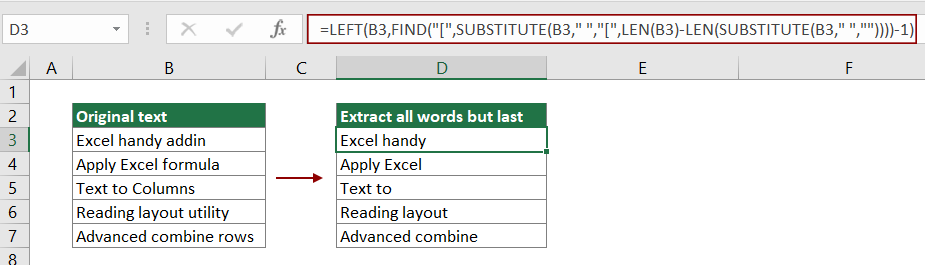Now all words in specific cells are extracted except the last words.

Note: In this above formula, B3 is the cell contains the words you will extract except the last one. Please change it based on your need.

How this formula works?

=LEFT(B3,FIND("[",SUBSTITUTE(B3," ","[",LEN(B3)-LEN(SUBSTITUTE(B3," ",""))))-1)

1. SUBSTITUTE(B3," ","[",LEN(B3)-LEN(SUBSTITUTE(B3," ","")))

• LEN(B3): The LEN function calculates the total length of text "Excel handy addin" and returns the result as 17;
• LEN(SUBSTITUTE(B3," ","")): The SUBSTITUTE function removes all spaces in "Excel handy addin" and returns "Excelhandyaddin", and then the LEN function calculates the total length of text "Excelhandyaddin". The final result is 15;
• SUBSTITUTE(B3," ","[",17-15)): SUBSTITUTE(B3," ","[",2)) The SUBSTITUTE function replaces the second space in "Excel handy addin" with a character "[" and returns the result as "Excel handy[addin".

• The FIND function locates the position of the character "[" in text string "Excel handy[addin". Here the character "[" is in the 12th position in the text string, so it returns the result as 12;

3. =LEFT(B3,12-1)

• The LEFT function extracts 11 characters (12-1=11) from the left side of the text string "Excel handy addin". The result is "Excel handy".

#### Related functions

Excel RIGHT function
The Excel RIGHT function extracts a specific number of characters from the right side of the text string.

Excel LEN function
The Excel LEN function returns the number of characters in a text string.

Excel FIND function
The Excel FIND function finds a string within another string, and returns the starting position of the string inside another one.

Excel LEFT function
The Excel LEFT function extracts the given number of characters from the left side of a supplied string.

Excel SUBSTITUTE function
The Excel SUBSTITUTE function replaces text or characters within a text string with another text or characters.

#### Related formulas

Extract Multiple Lines From A Cell
If you have a list of text strings which are separated by line breaks (that occurs by pressing Alt + Enter keys when entering the text), and want to extract these lines of text into multiple cells, the formula in this tutorial will do you a favor.

Extract Last Line Of Text From A Multi-Line Cell
To extract the last line of text from a text string which is separated by line breaks, a formula in this tutorial can help you deal with this task in Excel.

Extract Nth Word From Text String In Excel
This article explains how to use a formula to extract the specific nth word from a text string in a cell in Excel.

Extract Last Two Words From A Cell In Excel
This tutorial explains how to use formula to extract the last two words from a cell in Excel.

Extract Word Beginning With A Specific Character In Excel
This tutorial provides a formula with detail steps to help you extract the word which begins with a specific character from a text string in a cell in Excel.

### The Best Office Productivity Tools

#### Kutools for Excel - Helps You To Stand Out From Crowd

 Popular Features: Find/Highlight/Identify Duplicates  |  Delete Blank Rows  |  Combine Columns or Cells without Losing Data  |  Round without Formula ... Super VLookup: Multiple Criteria  |  Multiple Value   |  Across Multi-Sheets  |  Fuzzy Lookup... Adv. Drop-down List: Easy Drop Down List  |  Dependent Drop Down List  |  Multi-select Drop Down List... Column Manager: Add a Specific Number of Columns  |  Move Columns  |  Unhide Columns  |  Compare Columns to Select Same & Different Cells ... Featured Features: Grid Focus  |  Design View  |  Big Formula Bar  |  Workbook & Sheet Manager  |  Resource Library (Auto Text)  |  Date Picker  |  Combine Worksheets  |  Encrypt/Decrypt Cells  |  Send Emails by List  |  Super Filter  |  Special Filter (filter bold/italic/strikethrough...) ... Top 15 Toolsets:  12 Text Tools (Add Text, Remove Characters ...)  |  50+ Chart Types (Gantt Chart ...)  |  40+ Practical Formulas (Calculate age based on birthday ...)  |  19 Insertion Tools (Insert QR Code, Insert Picture from Path ...)  |  12 Conversion Tools (Numbers to Words, Currency Conversion ...)  |  7 Merge & Split Tools (Advanced Combine Rows, Split Cells ...)  |  ... and more

Kutools for Excel Boasts Over 300 Features, Ensuring That What You Need is Just A Click Away...

Supports Office/Excel 2007-2021 and 365   |   Available in 44 Languages   |   Easy to Uninstall Completely#### Office Tab - Enable Tabbed Reading and Editing in Microsoft Office (include Excel)

• One second to switch between dozens of open documents!
• Reduce hundreds of mouse clicks for you every day, say goodbye to mouse hand.
• Increases your productivity by 50% when viewing and editing multiple documents.
• Brings Efficient Tabs to Office (include Excel), Just Like Chrome, Edge and Firefox.